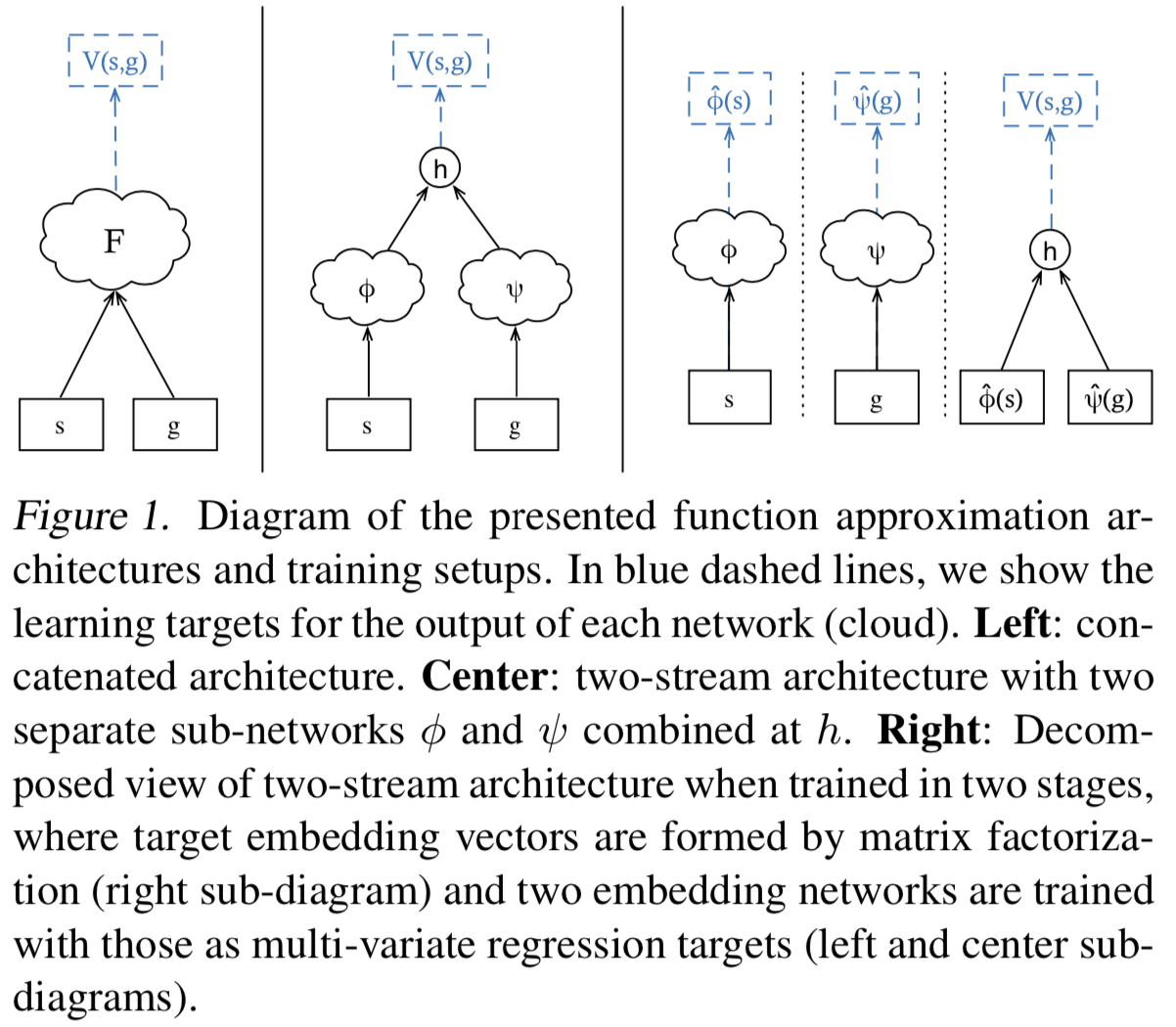• 文中理论说明很多，很晦涩，可以不看，直接跳至正文部分即可
• 思想简单，了解一下即可

# 简介

Our main idea is to represent a large set of optimal value functions by a single, unified function approximator that generalises over both states and goals.

# 文中正文

• 如何通用表示各种问题的值函数逼近器？

• 用单个函数逼近器表示多目标的最优值函数

• 算法的输入由状态$s$扩展为状态-目标$\lt s,g \gt$，假设原状态表示向量$s$不包含目标信息

• 直接用$||$连接，即$\left ( s||g \right )$，然后通过非线性函数逼近器(如MLPs)得到最终输出结果$V(s, g)$
• 分别embedding，并将嵌入后的表示通过运算得到最终输出结果(文中使用的是点积)，$h : \mathbb{R}^{n} \times \mathbb{R}^{n} \mapsto \mathbb{R}$，然后$V(s, g) :=h(\phi(s), \psi(g))$• UVFA可用于同任务多目标的迁移学习中，可以比随机值初始化更快地学习解决新任务。这是显而易见的作用，毕竟本身就是多目标的通用函数逼近器。
• 可以用于特征表示。这也说，= =，强行增加字数。。。。。。
• UVFA有效地提供了一个通用决策模型，代表（近似）朝向任何目标$g \in \mathcal{G}$的最佳行为。这个优点与现在提出的元强化学习中的slow部分如出一辙。

# 总结

UVFA把$V(s)$变成$V(s,g)$，一个可表示单任务、多目标的通用值函数近似，扩展对任务的知识表达。(通用即知识，哈哈)

-------------本文结束感谢您的阅读-------------

0%